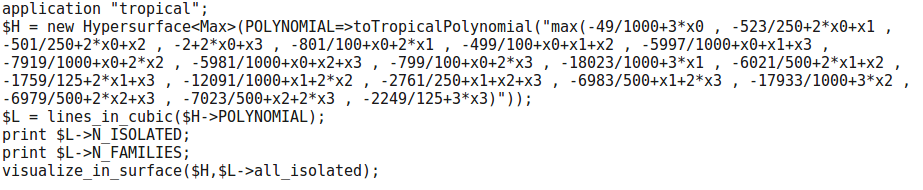# Problem 12¶

Give an example of a smooth tropical cubic surface in $$\mathbb{R}^3$$ that contains 27 distinct tropical lines. How many other lines does each line intersect? Can we draw a picture of that cubic surface together with the 27 lines?

## Solution¶

In Polymake:

application "tropical";
$H = new Hypersurface<Max>( POLYNOMIAL=>toTropicalPolynomial("max(-49/1000+3*x0 , -523/250+2*x0+x1 , -501/250+2*x0+x2 , -2+2*x0+x3 , -801/100+x0+2*x1 , -499/100+x0+x1+x2 , -5997/1000+x0+x1+x3 , -7919/1000+x0+2*x2 , -5981/1000+x0+x2+x3 , -799/100+x0+2*x3 , -18023/1000+3*x1 , -6021/500+2*x1+x2 , -1759/125+2*x1+x3 , -12091/1000+x1+2*x2 , -2761/250+x1+x2+x3 , -6983/500+x1+2*x3 , -17933/1000+3*x2 , -6979/500+2*x2+x3 , -7023/500+x2+2*x3 , -2249/125+3*x3)"));$L = lines_in_cubic($H->POLYNOMIAL); print$L->N_ISOLATED;
print $L->N_FAMILIES; visualize_in_surface($H,\$L->all_isolated);